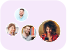Product Edition:2nd Edition
Author: Michael Baron
Book Name: Probability and Statistics for Computer Scientists
Subject Name: Maths

# Probability and Statistics for Computer Scientists 2nd Edition Solutions

0 out of 5.0
50 reviews35 Students
have requested for homework help from this book

Student-Friendly Coverage of Probability Statistical Methods Simulation and Modeling ToolsIncorporating feedback from instructors and researchers who used the previous edition Probability and Statistics for Computer Scientists Second Edition helps students understand general methods of stochastic modeling simulation and data analysismake optimal decisions under uncertaintymodel and evaluate computer systems and networksand prepare for advanced probability-based courses. Written in a lively style with simple language this classroom-tested book can now be used in both one- and two-semester courses.New to the Second EditionAxiomatic introduction of probabilityExpanded coverage of statistical inference including standard errors of estimates and their estimation inference about variances chi-square tests for independence and goodness of fit nonparametric statistics and bootstrapMore exercises at the end of each chapterAdditional MATLAB� codes particularly new commands of the Statistics ToolboxIn-Depth yet Accessible Treatment of Computer Science-Related TopicsStarting with the fundamentals of probability the text takes students through topics heavily featured in modern computer science computer engineering software engineering and associated fields such as computer simulations Monte Carlo methods stochastic processes Markov chains queuing theory statistical inference and regression. It also meets the requirements of the Accreditation Board for Engineering and Technology (ABET).Encourages Practical Implementation of SkillsUsing simple MATLAB commands (easily translatable to other computer languages) the book provides short programs for implementing the methods of probability and statistics as well as for visualizing randomness the behavior of random variables and stochastic processes convergence results and Monte Carlo simulations. Preliminary knowledge of MATLAB is not required. Along with numerous computer science applications and worked examples the text presents interesting facts and paradoxical statements. Each chapter concludes with a short summary and many exercises.Read more

5
25
4
10
3
2
2
2
1
11

0

## Students who viewed this book also checked out